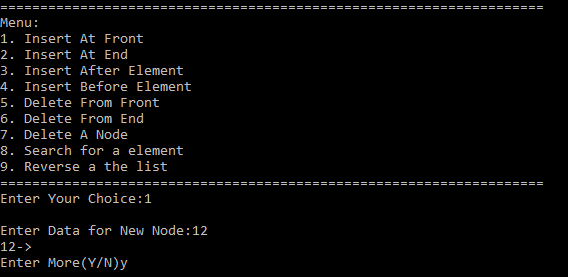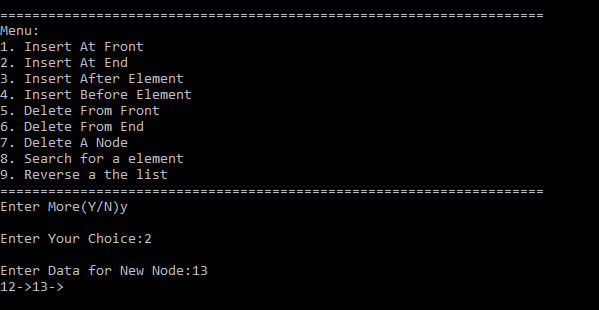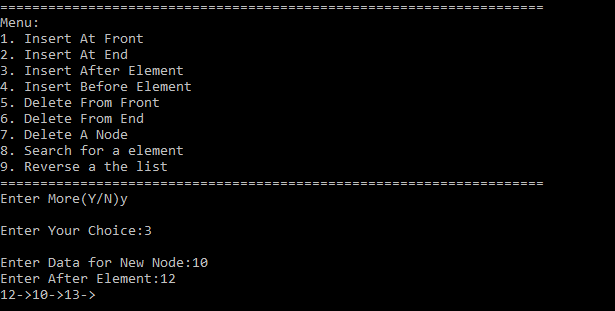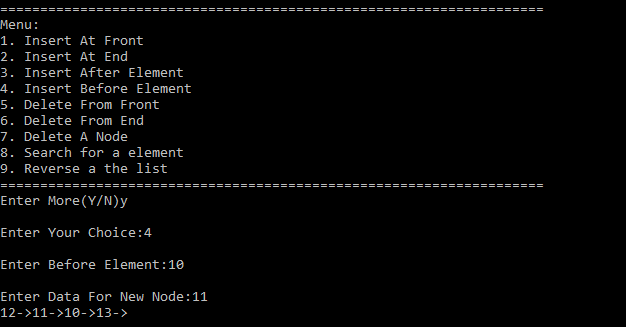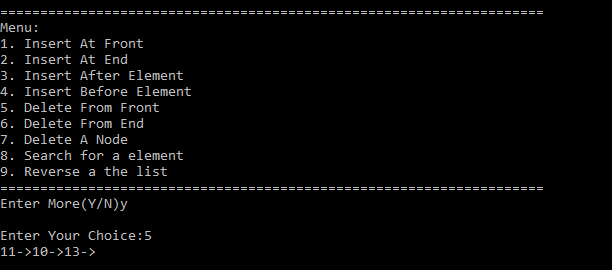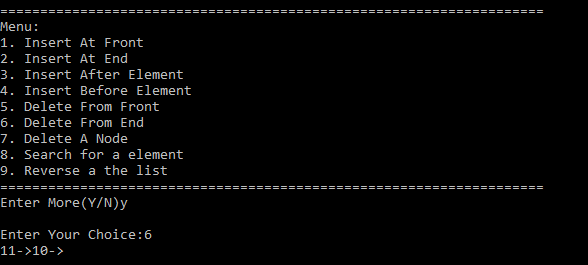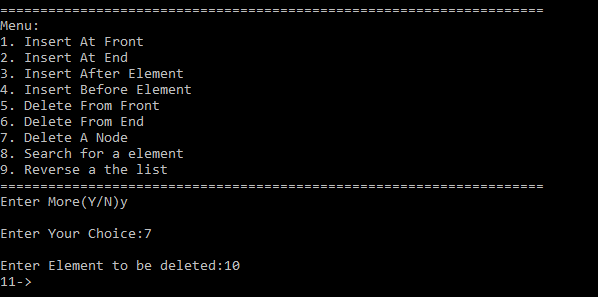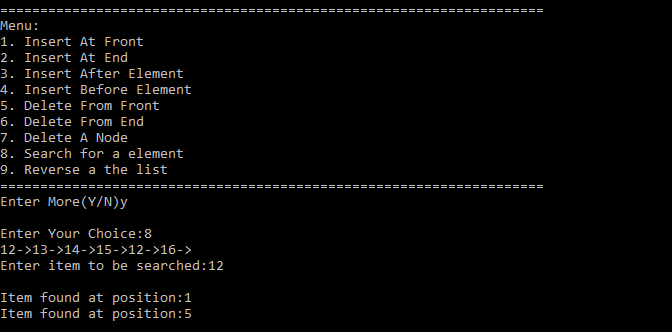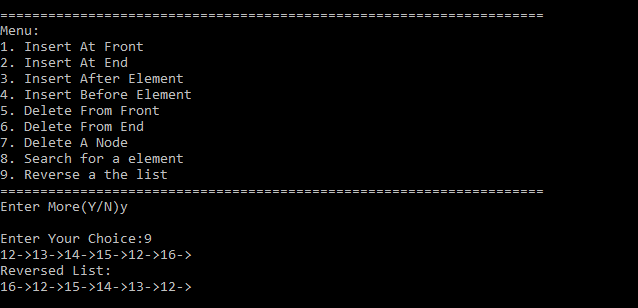Open in App
Not now

# Menu Driven Program to implement all the operations of Doubly Circular Linked List

• Last Updated : 28 Dec, 2022

Circular Doubly Linked List has properties of both Doubly Linked List and Circular Linked List in which two consecutive elements are linked or connected by previous and next pointer and the last node points to the first node by next pointer and also the first node points to the last node by the previous pointer. The program implements all the functions and operations that are possible on a doubly circular list following functions in a menu-driven program.

Approach: Each function (except display) takes the address of the pointer to the head i.e. the first element of the list. This allows us to change the address of the head pointer. The idea is the use only one pointer, which is of type node, to perform all the operations. node is the class that contains three data members. One member is of type int which stores data of the node and two members of type node*, one store’s address of next element and other stores address of the previous element. This way it is possible to traverse the list in either direction. It is possible to modify, insert, delete search for the element using a single pointer.

Functions Covered:

• void insert_front (node **head): This function allows the user to enter a new node at the front of the list.
• void insert_end (node **head): This function allows the user to enter a new node at the end of the list.
• void inser_after (node **head): This function allows the user to enter a new node after a node is entered by the user.
• void insert_before (node **head): This function allows the user to enter a new node before a node is entered by the user.
• void delete_front (node **head): This function allows the user to delete a  node from the front of the list.
• void delete_end(node**head): This function allows the user to delete a node from the end of the list.
• void delete_mid( node **head): This function allows deletion of a node entered by the user.
• void search (node *head): Function to search for the location of the array entered by the user.
• void reverse (node **head): Function to reverse the list and make the last element of the list head.
• void display (node *head): Function to print the list.

Below is the C++ program to implement the above approach:

## C++

 `// C++ program for the above approach``#include ``using` `namespace` `std;` `class` `node {``public``:``    ``node* next;``    ``node* prev;``    ``int` `data;``};` `// Function to add the node at the front``// of the doubly circular LL``void` `insert_front(node** head)``{``    ``// Function to insert node``    ``// in front of list``    ``cout << ``"\nEnter Data for New Node:"``;` `    ``// Create a new node named``    ``// new_node``    ``node* new_node = ``new` `node;` `    ``// Enter data for new_node``    ``cin >> new_node->data;` `    ``if` `(*head == NULL) {` `        ``// If there is no node in``        ``// the list, create a node``        ``// pointing to itself and``        ``// make it head``        ``new_node->next = new_node;``        ``new_node->prev = new_node;``        ``*head = new_node;``    ``}` `    ``else` `{` `        ``// If there already exists``        ``// elements in the list` `        ``// Next of new_node will point``        ``// to head``        ``new_node->next = *head;` `        ``// prev of new_node will point``        ``// to prev of head``        ``new_node->prev = (*head)->prev;` `        ``// next of prev of head i.e. next``        ``// of last node will point to``        ``// new_node``        ``((*head)->prev)->next = new_node;` `        ``// prev of head will point``        ``// to new_node``        ``(*head)->prev = new_node;` `        ``// new_node will become the``        ``// head of list``        ``*head = new_node;``    ``}``}` `// Function to add the node at the end``// of the doubly circular LL``void` `insert_end(node** head)``{``    ``// Function to insert node at``    ``// last of list``    ``cout << ``"\nEnter Data for New Node:"``;` `    ``// Create new node``    ``node* new_node = ``new` `node;``    ``cin >> new_node->data;` `    ``if` `(*head == NULL) {` `        ``// If there is no element in the``        ``// list create a node pointing``        ``// to itself and make it head``        ``new_node->next = new_node;``        ``new_node->prev = new_node;``        ``*head = new_node;``    ``}``    ``else` `{` `        ``// If there are elements in the``        ``// list then create a temp node``        ``// pointing to current element``        ``node* curr = *head;` `        ``while` `(curr->next != *head)` `            ``// Traverse till the end of``            ``// list``            ``curr = curr->next;` `        ``// next of new_node will point to``        ``// next of current node``        ``new_node->next = curr->next;` `        ``// prev of new_node will``        ``// point to current element``        ``new_node->prev = curr;` `        ``// prev of next of current node``        ``// i.e. prev of head will point to``        ``// new_node``        ``(curr->next)->prev = new_node;` `        ``// next of current node will``        ``// point to new_node``        ``curr->next = new_node;``    ``}``}` `// Function to add the node after the``// given node of doubly circular LL``void` `insert_after(node** head)``{``    ``// Function to enter a node after``    ``// the element entered by user` `    ``// Create new node``    ``node* new_node = ``new` `node;``    ``cout << ``"\nEnter Data for New Node:"``;``    ``cin >> new_node->data;` `    ``if` `(*head == NULL) {` `        ``// If there is no element in``        ``// the list then create a node``        ``// pointing to itself and make``        ``// it head``        ``cout << ``"\nThere is No element in the List"``;``        ``cout << ``"\nCreating a new node"``;``        ``new_node->prev = new_node;``        ``new_node->next = new_node;``        ``*head = new_node;``    ``}``    ``else` `{``        ``int` `num;` `        ``// Ask user after which node new``        ``// node is to be inserted``        ``cout << ``"Enter After Element:"``;``        ``cin >> num;` `        ``// temp node to traverse list``        ``// and point to current element``        ``node* curr = *head;` `        ``while` `(curr->data != num) {``            ``curr = curr->next;` `            ``// If current becomes equal``            ``// to head i.e. if entire list``            ``// has been traversed then``            ``// element entered is not found``            ``// in list``            ``if` `(curr == *head) {` `                ``cout << ``"\nEntered Element"``                     ``<< ``" Not Found in "``                        ``"List\n"``;``                ``return``;``            ``}``        ``}` `        ``// Control will reach here only if``        ``// element is found in list` `        ``// next of new node will point to``        ``// next of current node``        ``new_node->next = curr->next;` `        ``// prev of new node will``        ``// point to current node``        ``new_node->prev = curr;` `        ``// prev of next of current node``        ``// will point to new node``        ``(curr->next)->prev = new_node;` `        ``// next of current node will``        ``// point to new node``        ``curr->next = new_node;``    ``}``}` `// Function to add the node before the``// given node of doubly circular LL``void` `insert_before(node** head)``{``    ``// Function to enter node before``    ``// a node entered by the user``    ``node* new_node = ``new` `node;` `    ``if` `(*head == NULL) {` `        ``// If there is no element in the``        ``// list create new node and make``        ``// it head``        ``cout << ``"List is Empty!! Creating New node..."``;``        ``cout << ``"\nEnter Data for New Node:"``;``        ``cin >> new_node->data;` `        ``new_node->prev = new_node;``        ``new_node->next = new_node;``        ``*head = new_node;``    ``}` `    ``else` `{``        ``int` `num;` `        ``// Ask user before which node``        ``// new node is to be inserted``        ``cout << ``"\nEnter Before Element:"``;``        ``cin >> num;` `        ``// If user wants to enter new node``        ``// before the first node i.e.``        ``// before head then call insert_front``        ``// function``        ``if` `((*head)->data == num)``            ``insert_front(head);` `        ``else` `{` `            ``// temp node current for traversing``            ``// the list and point to current``            ``// element we assign curr to``            ``// *head->next this time because``            ``// data of head has already been``            ``// checked in previous condition``            ``node* curr = (*head)->next;` `            ``while` `(curr->data != num) {``                ``if` `(curr == *head) {` `                    ``// If current equal head then``                    ``// entire list has been traversed``                    ``// and the entered element is not``                    ``// found in list``                    ``cout << ``"\nEntered Element Not Found "``                            ``"in List!!\n"``;``                    ``return``;``                ``}``                ``curr = curr->next;``            ``}` `            ``cout << ``"\nEnter Data For New Node:"``;``            ``cin >> new_node->data;` `            ``// Control will reaach here only``            ``// if entered node exists in list``            ``// and current has found the element` `            ``// next of new node will point to``            ``// current node``            ``new_node->next = curr;` `            ``// prev of new node will point``            ``// to prev of current node``            ``new_node->prev = curr->prev;` `            ``// next of prev of current node``            ``// will point to new node``            ``(curr->prev)->next = new_node;` `            ``// prev of current will``            ``// point to new node``            ``curr->prev = new_node;``        ``}``    ``}``}` `// Function to delete the front node``// of doubly circular LL``void` `delete_front(node** head)``{``    ``// Function to delete a node``    ``// from front of list``    ``if` `(*head == NULL) {` `        ``// If list is already empty``        ``// print a message``        ``cout << ``"\nList in empty!!\n"``;``    ``}``    ``else` `if` `((*head)->next == *head) {` `        ``// If head is the only element``        ``// in the list delete head and``        ``// assign it to NULL``        ``delete` `*head;``        ``*head = NULL;``    ``}``    ``else` `{``        ``node* curr = ``new` `node;` `        ``// temp node to save address``        ``// of node next to head``        ``curr = (*head)->next;` `        ``// prev of temp will``        ``// point to prev of head``        ``curr->prev = (*head)->prev;` `        ``// next of prev of head i.e.``        ``// next of last node will point``        ``// to temp``        ``((*head)->prev)->next = curr;` `        ``// delete head``        ``delete` `*head;` `        ``// assign head to temp``        ``*head = curr;``    ``}``}` `// Function to delete the end node``// of doubly circular LL``void` `delete_end(node** head)``{``    ``// Function to delete a node``    ``// from end of list``    ``if` `(*head == NULL) {` `        ``// If list is already empty``        ``// print a message``        ``cout << ``"\nList is Empty!!\n"``;``    ``}``    ``else` `if` `((*head)->next == *head) {` `        ``// If head is the only element``        ``// in the list delete head and``        ``// assign it to NULL``        ``delete` `*head;``        ``*head = NULL;``    ``}``    ``else` `{` `        ``// Create temporary node curr``        ``// to traverse list and point``        ``// to current element``        ``node* curr = ``new` `node;` `        ``// Assign curr to head``        ``curr = *head;``        ``while` `(curr->next != (*head)) {` `            ``// Traverse till end of list``            ``curr = curr->next;``        ``}` `        ``// next of prev of curr will point``        ``// to next of curr``        ``(curr->prev)->next = curr->next;` `        ``// prev of next of curr will point``        ``// to prev of curr``        ``(curr->next)->prev = curr->prev;` `        ``// delete curr``        ``delete` `curr;``    ``}``}` `// Function to delete the middle node``// of doubly circular LL``void` `delete_mid(node** head)``{``    ``// Function to delete a node``    ``// entered by user``    ``if` `(*head == NULL) {` `        ``// If list is already empty``        ``// print a message``        ``cout << ``"\nList is Empty!!!"``;``    ``}` `    ``else` `{``        ``cout << ``"\nEnter Element to be deleted:"``;``        ``int` `num;``        ``cin >> num;` `        ``if` `((*head)->data == num) {` `            ``// If user wants to delete``            ``// the head node i.e front``            ``// node call delete_front(head)``            ``// function``            ``delete_front(head);``        ``}` `        ``else` `{` `            ``// temp node to traverse list``            ``// and point to current node``            ``node* curr = (*head)->next;``            ``while` `((curr->data) != num) {``                ``if` `(curr == (*head)) {` `                    ``// If curr equals head then``                    ``// entire list has been``                    ``// traversed element to be``                    ``// deleted is not found``                    ``cout << ``"\nEntered Element Not Found "``                            ``"in List!!\n"``;``                    ``return``;``                ``}` `                ``curr = curr->next;``            ``}` `            ``// control will reach here only``            ``// if element is found in the list` `            ``// next of prev of curr will``            ``// point to next of curr``            ``(curr->prev)->next = curr->next;` `            ``// prev of next of curr will``            ``// point to prev of curr``            ``(curr->next)->prev = curr->prev;``            ``delete` `curr;``        ``}``    ``}``}` `// Function to search any node in the``// doubly circular LL``void` `search(node* head)``{``    ``if` `(head == NULL) {` `        ``// If head is null list is empty``        ``cout << ``"List is empty!!"``;``        ``return``;``    ``}` `    ``int` `item;``    ``cout << ``"Enter item to be searched:"``;` `    ``// Ask user to enter item to``    ``// be searched``    ``cin >> item;` `    ``// curr pointer is used to``    ``// traverse list it will point``    ``// to the current element``    ``node* curr = head;` `    ``int` `index = 0, count = 0;` `    ``do` `{` `        ``// If data in curr is equal to item``        ``// to be searched print its position``        ``// index+1``        ``if` `(curr->data == item) {``            ``cout << ``"\nItem found at position:"``                 ``<< index + 1;` `            ``// increment count``            ``count++;``        ``}` `        ``// Index will increment by 1 in``        ``// each iteration``        ``index++;``        ``curr = curr->next;` `    ``} ``while` `(curr != head);` `    ``// If count is still 0 that means``    ``// item is not found``    ``if` `(count == 0)``        ``cout << ``"Item searched not found in list"``;``}` `// Function to reverse the doubly``// circular Linked List``void` `reverse(node** head)``{``    ``if` `(*head == NULL) {` `        ``// If head is null list is empty``        ``cout << ``"List is Empty !!"``;``        ``return``;``    ``}` `    ``// curr is used to traverse list``    ``node* curr = *head;``    ``while` `(curr->next != *head) {` `        ``// use a temp node to store``        ``// address of next of curr``        ``node* temp = curr->next;` `        ``// make next of curr to point``        ``// its previous``        ``curr->next = curr->prev;` `        ``// make previous of curr to``        ``// point its next``        ``curr->prev = temp;` `        ``// After each iteration move``        ``// to element which was earlier``        ``// next of curr``        ``curr = temp;``    ``}` `    ``// Update the last node separately``    ``node* temp = curr->next;``    ``curr->next = curr->prev;``    ``curr->prev = temp;` `    ``// only change is this node will now``    ``// become head``    ``*head = curr;``}` `// Function to display the doubly``// circular linked list``void` `display(node* head)``{``    ``node* curr = head;``    ``if` `(curr == NULL)``        ``cout << ``"\n List is Empty!!"``;``    ``else` `{``        ``do` `{``            ``cout << curr->data << ``"->"``;``            ``curr = curr->next;``        ``} ``while` `(curr != head);``    ``}``}` `void` `display_menu()``{``    ``cout << ``"=============================================="``            ``"======================"``;``    ``cout << ``"\nMenu:\n"``;``    ``cout << ``"1. Insert At Front\n"``;``    ``cout << ``"2. Insert At End\n"``;``    ``cout << ``"3. Insert After Element\n"``;``    ``cout << ``"4. Insert Before Element\n"``;``    ``cout << ``"5. Delete From Front\n"``;``    ``cout << ``"6. Delete From End\n"``;``    ``cout << ``"7. Delete A Node\n"``;``    ``cout << ``"8. Search for a element\n"``;``    ``cout << ``"9. Reverse a the list\n"``;``    ``cout << ``"=============================================="``            ``"======================"``;``}` `// Driver Code``int` `main()``{``    ``int` `choice;``    ``char` `repeat_menu = ``'y'``;` `    ``// Declaration of head node``    ``node* head = NULL;``    ``display_menu();``    ``do` `{``        ``cout << ``"\nEnter Your Choice:"``;``        ``cin >> choice;``        ``switch` `(choice) {``        ``case` `1: {``            ``insert_front(&head);``            ``display(head);``            ``break``;``        ``}``        ``case` `2: {``            ``insert_end(&head);``            ``display(head);``            ``break``;``        ``}``        ``case` `3: {``            ``insert_after(&head);``            ``display(head);``            ``break``;``        ``}``        ``case` `4: {``            ``insert_before(&head);``            ``display(head);``            ``break``;``        ``}``        ``case` `5: {``            ``delete_front(&head);``            ``display(head);``            ``break``;``        ``}``        ``case` `6: {``            ``delete_end(&head);``            ``display(head);``            ``break``;``        ``}``        ``case` `7: {``            ``delete_mid(&head);``            ``display(head);``            ``break``;``        ``}``        ``case` `8: {``            ``search(head);``            ``break``;``        ``}``        ``case` `9: {``            ``reverse(&head);``            ``display(head);``            ``break``;``        ``}``        ``default``: {``            ``cout << ``"\nWrong Choice!!!"``;``            ``display_menu();``            ``break``;``        ``}``        ``}``        ``cout << ``"\nEnter More(Y/N)"``;``        ``cin >> repeat_menu;` `    ``} ``while` `(repeat_menu == ``'y'` `|| repeat_menu == ``'Y'``);``    ``return` `0;``}`

## Python3

 `# Python program for the above approach` `class` `Node:``    ``def` `__init__(``self``,data):``        ``self``.data ``=` `data``        ``self``.prev ``=` `None``        ``self``.``next` `=` `None``        ` `#  Function to add the node at the front``#  of the doubly circular LL``def` `insert_front(head):``    ``# Function to insert node in front of list``    ``print``(``"Enter Data for New Node: "``)` `    ``# Create a new node named new_node``    ``new_node ``=` `Node(``int``(``input``()))``    ` `    ``if` `head ``=``=` `None``:``        ``# If there is no node in``        ``# the list, create a node``        ``# pointing to itself and``        ``# make it head``        ``new_node.``next` `=` `new_node``        ``new_node.prev ``=` `new_node``        ``head ``=` `new_node``    ``else``:``        ``# If there already exists``        ``# elements in the list``        ``# Next of new_node will point``        ``# to head``        ``new_node.``next` `=` `head``        ` `        ``# prev of new_node will point``        ``# to prev of head``        ``new_node.prev ``=` `head.prev``        ` `        ``# next of prev of head i.e. next``        ``# of last node will point to``        ``# new_node``        ``new_node.prev.``next` `=` `new_node``        ` `        ``#  prev of head will point``        ``#  to new_node``        ``head.prev ``=` `new_node` `        ``# new_node will become the``        ``# head of list``        ``head ``=` `new_node` `# Function to add the node at the end``# of the doubly circular LL``def` `insert_end(head):``    ``# Function to insert node at``    ``# last of list``    ``print``(``"Enter Data for New Node: "``)` `    ``# Create new node``    ``new_node ``=` `None``(``int``(``input``()))` `    ``if` `(head ``=``=` `None``):``        ``# If there is no element in the``        ``# list create a node pointing``        ``# to itself and make it head``        ``new_node.``next` `=` `new_node``        ``new_node.prev ``=` `new_node``        ``head ``=` `new_node``    ``else``:``        ``# If there are elements in the``        ``# list then create a temp node``        ``# pointing to current element``        ``curr ``=` `head` `        ``while` `(curr.``next` `!``=` `head):``            ``# Traverse till the end of``            ``# list``            ``curr ``=` `curr.``next` `        ``# next of new_node will point to``        ``# next of current node``        ``new_node.``next` `=` `curr.``next` `        ``# prev of new_node will``        ``# point to current element``        ``new_node.prev ``=` `curr` `        ``# prev of next of current node``        ``# i.e. prev of head will point to``        ``# new_node``        ``curr.``next``.prev ``=` `new_node` `        ``# next of current node will``        ``# point to new_node``        ``curr.``next` `=` `new_node` `# Function to add the node after the``# given node of doubly circular LL``def` `insert_after(head):``    ``# Function to enter a node after``    ``# the element entered by user` `    ``# Create new node``    ``new_node ``=` `Node(``int``(``input``(``"Enter Data for New Node: "``)))``    ` `    ``if` `(head ``=``=` `None``):``        ``# If there is no element in``        ``# the list then create a node``        ``# pointing to itself and make``        ``# it head``        ``print``(``"There is No element in the List"``)``        ``print``(``"Creating a new node"``)``        ``new_node.prev ``=` `new_node``        ``new_node.``next` `=` `new_node``        ``head ``=` `new_node``    ``else``:``        ``# Ask user after which node new``        ``# node is to be inserted``        ``num ``=` `int``(``input``(``"Enter After Element:"``))` `        ``# temp node to traverse list``        ``# and point to current element``        ``curr ``=` `head` `        ``while` `(curr.data !``=` `num):``            ``curr ``=` `curr.``next` `            ``# If current becomes equal``            ``# to head i.e. if entire list``            ``# has been traversed then``            ``# element entered is not found``            ``# in list``            ``if` `(curr ``=``=` `head):``                ``print``(``"Entered Element Not Found in List"``)``                ``return` `        ``# Control will reach here only if``        ``# element is found in list` `        ``# next of new node will point to``        ``# next of current node``        ``new_node.``next` `=` `curr.``next` `        ``# prev of new node will``        ``# point to current node``        ``new_node.prev ``=` `curr` `        ``# prev of next of current node``        ``# will point to new node``        ``curr.``next``.prev ``=` `new_node` `        ``# next of current node will``        ``# point to new node``        ``curr.``next` `=` `new_node`` `  `# Function to add the node before the``# given node of doubly circular LL``def` `insert_before(head):``    ``# Function to enter node before``    ``# a node entered by the user` `    ``if` `(head ``=``=` `None``) :` `        ``# If there is no element in the``        ``# list create new node and make``        ``# it head``        ``print``(``"List is Empty!! Creating New node..."``)``        ``new_node ``=` `Node(``int``(``input``(``"Enter Data for New Node:"``)))` `        ``new_node.prev ``=` `new_node``        ``new_node.``next` `=` `new_node``        ``head ``=` `new_node ``    ``else` `:``        ``# Ask user before which node``        ``# new node is to be inserted``        ``num ``=` `int``(``input``(``"Enter Before Element: "``))` `        ``# If user wants to enter new node``        ``# before the first node i.e.``        ``# before head then call insert_front``        ``# function``        ``if` `(head.data ``=``=` `num):``            ``insert_front(head)``        ``else` `:``            ``# temp node current for traversing``            ``# the list and point to current``            ``# element we assign curr to``            ``# head.next this time because``            ``# data of head has already been``            ``# checked in previous condition``            ``curr ``=` `head.``next` `            ``while` `(curr.data !``=` `num):``                ``if` `(curr ``=``=` `head):` `                    ``# If current equal head then``                    ``# entire list has been traversed``                    ``# and the entered element is not``                    ``# found in list``                    ``print``(``"Entered Element Not Found in List!!"``)``                    ``return`                `                ``curr ``=` `curr.``next``             ` `            ``new_node ``=` `Node(``int``(``input``(``"Enter Data For New Node: "``)))` `            ``# Control will reaach here only``            ``# if entered node exists in list``            ``# and current has found the element` `            ``# next of new node will point to``            ``# current node``            ``new_node.``next` `=` `curr` `            ``# prev of new node will point``            ``# to prev of current node``            ``new_node.prev ``=` `curr.prev` `            ``# next of prev of current node``            ``# will point to new node``            ``curr.prev.``next` `=` `new_node` `            ``# prev of current will``            ``# point to new node``            ``curr.prev ``=` `new_node``       ` `         ` `# Function to delete the front node``# of doubly circular LL``def` `delete_front(head):``    ``# Function to delete a node``    ``# from front of list``    ``if` `(head ``=``=` `None``):``        ``# If list is already empty``        ``# print a message``        ``print``(``"List in empty!!"``)``     ` `    ``elif` `(head.``next` `=``=` `head):``        ``# If head is the only element``        ``# in the list delete head and``        ``# assign it to None``        ``head ``=` `None``     ` `    ``else` `:``        ``# temp node to save address``        ``# of node next to head``        ``curr ``=` `head.``next` `        ``# prev of temp will``        ``# point to prev of head``        ``curr.prev ``=` `head.prev` `        ``# next of prev of head i.e.``        ``# next of last node will point``        ``# to temp``        ``head.prev.``next` `=` `curr` `        ``# delete head``        ``head ``=` `None` `        ``# assign head to temp``        ``head ``=` `curr``     `  `# Function to delete the end node``# of doubly circular LL``def` `delete_end(head):``    ``# Function to delete a node``    ``# from end of list``    ``if` `(head ``=``=` `None``):` `        ``# If list is already empty``        ``# print a message``        ``print``(``"List is Empty!!"``)``     ` `    ``elif` `(head.``next` `=``=` `head) :` `        ``# If head is the only element``        ``# in the list delete head and``        ``# assign it to None``        ``head ``=` `None``     ` `    ``else` `:` `        ``# Create temporary node curr``        ``# to traverse list and point``        ``# to current element` `        ``# Assign curr to head``        ``curr ``=` `head``        ``while` `(curr.``next` `!``=` `head) :` `            ``# Traverse till end of list``            ``curr ``=` `curr.``next``         `  `        ``# next of prev of curr will point``        ``# to next of curr``        ``(curr.prev).``next` `=` `curr.``next` `        ``# prev of next of curr will point``        ``# to prev of curr``        ``(curr.``next``).prev ``=` `curr.prev` `        ``# delete curr``        ``curr ``=` `None``     ` ` `  `# Function to delete the middle node``# of doubly circular LL``def` `delete_mid(head):``    ``# Function to delete a node``    ``# entered by user``    ` `    ``if` `(head ``=``=` `None``):``        ``# If list is already empty``        ``# print a message``        ``print``(``"List is Empty!!!"``)``    ``else``:``        ``num ``=` `int``(``input``(``"Enter Element to be deleted: "``))` `        ``if` `(head.data ``=``=` `num):` `            ``# If user wants to delete``            ``# the head node i.e front``            ``# node call delete_front(head)``            ``# function``            ``delete_front(head)``        ``else``:``            ``# temp node to traverse list``            ``# and point to current node``            ``curr ``=` `head.``next``            ``while` `(curr.data !``=` `num) :``                ``if` `(curr ``=``=` `head):` `                    ``# If curr equals head then``                    ``# entire list has been``                    ``# traversed element to be``                    ``# deleted is not found``                    ``print``(``"Entered Element Not Found in List!!"``)``                    ``return``                ``curr ``=` `curr.``next``        ` `            ``# control will reach here only``            ``# if element is found in the list` `            ``# next of prev of curr will``            ``# point to next of curr``            ``curr.prev.``next` `=` `curr.``next` `            ``# prev of next of curr will``            ``# point to prev of curr``            ``curr.``next``.prev ``=` `curr.prev``            ``curr ``=` `None``         ` `     ` `# Function to search any node in the``# doubly circular LL``def` `search(head):``    ``if` `(head ``=``=` `None``) :``        ``# If head is None list is empty``        ``print``(``"List is empty!!"``)``        ``return`      `    ``# Ask user to enter item to``    ``# be searched``    ``item ``=` `int``(``input``(``"Enter item to be searched: "``))` `    ``# curr pointer is used to``    ``# traverse list it will point``    ``# to the current element``    ``curr ``=` `head.``next` `    ``index ``=` `0``    ``count ``=` `0``    ``if` `item ``=``=` `head.data:``        ``print``(``"Item found at position:"``,``1``)``        ` `    ``while` `(curr !``=` `head):``        ``# If data in curr is equal to item``        ``# to be searched print its position``        ``# index+1``        ``if` `(curr.data ``=``=` `item) :``            ``print``(``"Item found at position:"``,index ``+` `1``)``            ``# increment count``            ``count ``+``=` `1` `        ``# Index will increment by 1 in``        ``# each iteration``        ``index ``+``=` `1``        ``curr ``=` `curr.``next`  `    ``# If count is still 0 that means``    ``# item is not found``    ``if` `(count ``=``=` `0``):``        ``print``(``"Item searched not found in list"``)`` `  `# Function to reverse the doubly``# circular Linked List``def` `reverse(head):``    ``if` `(head ``=``=` `None``):``        ``# If head is None list is empty``        ``print``(``"List is Empty !!"``)``        ``return`      `    ``# curr is used to traverse list``    ``curr ``=` `head``    ``while` `(curr.``next` `!``=` `head):` `        ``# use a temp node to store``        ``# address of next of curr``        ``temp ``=` `curr.``next` `        ``# make next of curr to point``        ``# its previous``        ``curr.``next` `=` `curr.prev` `        ``# make previous of curr to``        ``# point its next``        ``curr.prev ``=` `temp` `        ``# After each iteration move``        ``# to element which was earlier``        ``# next of curr``        ``curr ``=` `temp``     `  `    ``# Update the last node separately``    ``temp ``=` `curr.``next``    ``curr.``next` `=` `curr.prev``    ``curr.prev ``=` `temp` `    ``# only change is this node will now``    ``# become head``    ``head ``=` `curr`` `  `# Function to display the doubly``# circular linked list``def` `display(head):``    ``curr ``=` `head.``next``    ``if` `(curr ``=``=` `None``):``        ``print``(``"List is Empty!!"``)``    ``else` `:``        ``print``(head.data,``'.'``)``        ``while` `(curr !``=` `head):``            ``print``(curr.data,``"."``)``            ``curr ``=` `curr.``next``     `  `def` `display_menu():``    ``print``(``"===================================================================="``)``    ``print``(``"Menu:"``)``    ``print``(``"1. Insert At Front"``)``    ``print``(``"2. Insert At End"``)``    ``print``(``"3. Insert After Element"``)``    ``print``(``"4. Insert Before Element"``)``    ``print``(``"5. Delete From Front"``)``    ``print``(``"6. Delete From End"``)``    ``print``(``"7. Delete A Node"``)``    ``print``(``"8. Search for a element"``)``    ``print``(``"9. Reverse a the list"``)``    ``print``(``"===================================================================="``)` `# Driver Code``choice ``=` `0``repeat_menu ``=` `'y'` `# Declaration of head node``head ``=` `None``display_menu()``while` `(repeat_menu ``=``=` `'y'` `or` `repeat_menu ``=``=` `'Y'``):``    ``choice ``=` `int``(``input``(``"Enter Your Choice: "``))``    ``if` `choice ``=``=` `1``:``        ``insert_front(head)``        ``display(head)``    ``elif` `choice ``=``=` `2``:``        ``insert_end(head)``        ``display(head)``    ``elif` `choice ``=``=` `3``:``        ``insert_after(head)``        ``display(head)``    ``elif` `choice ``=``=` `4``:``        ``insert_before(head)``        ``display(head)``    ``elif` `choice ``=``=` `5``:``        ``delete_front(head)``        ``display(head)``    ``elif` `choice ``=``=` `6``:``        ``delete_end(head)``        ``display(head)``    ``elif` `choice ``=``=` `7``:``        ``delete_mid(head)``        ``display(head)``    ``elif` `choice ``=``=` `8``:``        ``search(head)``    ``elif` `choice ``=``=` `9``:``        ``reverse(head)``        ``display(head)``    ``else``:``        ``print``(``"Wrong Choice!!!"``)``        ``display_menu()``    ``repeat_menu  ``=` `input``(``"Enter More(Y/N)"``)`

Output: